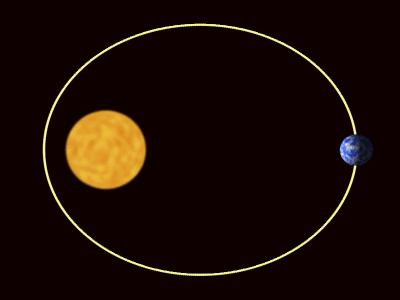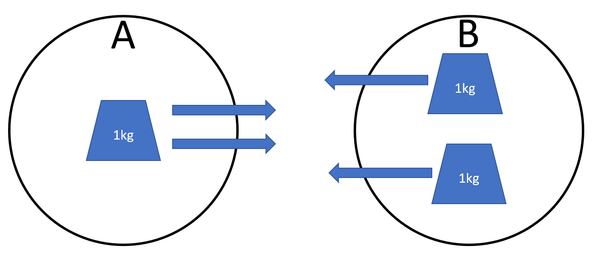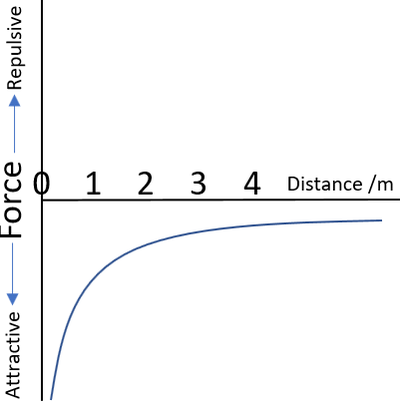# Gravity

## Key Stage 2

### Meaning

Gravity is a force that causes all objects to be attracted to each other.

Gravity is a non-contact force.
Gravity affects all objects.
Gravity pulls us down towards the centre of the Earth.If person A drops the cog it will fall towards the centre of the Earth. If person B drops the spanner it will fall towards the centre of the Earth.
Gravity is what keeps the Earth orbiting The Sun.The planets are pulled towards the The Sun by gravity.

## Key Stage 3

### Meaning

Gravity is a force that causes all objects with mass to be attracted to each other.

Gravity is a force so it is measured in Newtons.
Gravity is a non-contact force because it can act without objects touching.
All objects are affected by gravity.
Gravity is the force that pulls objects to the ground.
Isaac Newton was the first to realise that gravity is the force that holds the planets in orbit around The Sun.
The force of gravity between two objects is always equal in magnitude but opposite in direction.Object A has 1kg of mass and is attracted to object B. Object B has 2kg of mass and is attracted to object A. The force acting on object A is the same as the force on object B.

### Falling

#### Energy Transfers

##### Accelerating
When an object accelerates towards the ground energy is transferred from the gravitational potential energy store of the object to the kinetic energy store of the object.
##### Decelerating
When an object decelerates away from the ground energy is transferred from the kinetic energy store of the object to the gravitational potential energy store of the object.

#### Forces

##### Accelerating
When an object accelerates towards the ground gravity is causing a mechanical energy transfer.
##### Decelerating
When an object decelerates away from the ground gravity is causing a mechanical energy transfer.

### Orbits

Newton was the first person to realise that objects were held in orbit by gravity which he explained in his Law of Universal Gravitation.
Moons orbit planets and planets orbit the stars due to gravity.
Gravity is a constant force directed to the centre of a massive object.
The Moon feels a force pulling it towards the centre of the Earth. The Earth feels a force equal in magnitude but opposite in direction to that of The Moon.The planets are pulled towards The Sun by gravity.

## Key Stage 4

### Meaning

Gravity is a force of attraction which acts between all objects with mass.

The strength of gravity between two objects depends on:

When a small mass object is in the Gravitational Field of a large mass object the force on the small mass is referred to as weight rather than gravity.Object A has 1kg of mass and is attracted to object B. Object B has 2kg of mass and is attracted to object A. The force acting on object A is the same as the force on object B.

### Falling

#### Energy Transfers

##### Accelerating
When an object accelerates towards the ground energy is transferred from the gravitational potential energy store of the object to the kinetic energy store of the object.
##### Decelerating
When an object decelerates away from the ground energy is transferred from the kinetic energy store of the object to the gravitational potential energy store of the object.

#### Forces

##### Accelerating
When an object accelerates towards the ground gravity is causing a mechanical energy transfer.
##### Decelerating
When an object decelerates away from the ground gravity is causing a mechanical energy transfer.

### Orbits

Newton was the first person to realise that objects were held in orbit by gravity which he explained in his Law of Universal Gravitation.
Moons orbit planets and planets orbit the stars due to gravity.
Gravity is a constant force directed to the centre of a massive object.
The Moon feels a force pulling it towards the centre of the Earth. The Earth feels a force equal in magnitude but opposite in direction to that of The Moon.The planets are pulled towards The Sun by gravity.

## Key Stage 5

### Meaning

The gravitational interaction is the mechanism by which all particles with mass affect one another.

### About The Gravitational Interaction at Subatomic Scales

The gravitational interaction is one of the 4 fundamental interactions governing how particles affect one another.
The gravitational interaction causes all particles with mass to be attracted to one another.
The gravitational interaction is extremely weak on the scale of atomic masses but is 'long range' and cannot be shielded, so accumulates with large numbers of atoms to be observable at macroscopic scales.
The gravitational interaction may be mediated by an, as yet, undiscovered fundamental particle called a graviton.

### About The Gravitational Interaction at Macroscopic Scales

Due to its long range and the inability to shield the gravitational interaction it is responsible for the large scale structure of the observable universe.This graph shows the strength of the force between two objects with mass. The gravitational interaction between masses is attractive at a range of 0 to ∞. The strength of the force reduces proportional to 1/r2 where r is the distance between the centre of mass of each object.